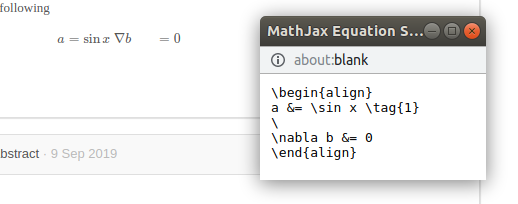Several LaTeX issues (fix suggested)

I use Indico for Math conference, and need the most of LaTeX math the system can produce.
I noticed several issues with it.

1. In the input page of abstract, I use multiline formula, like
\begin{align} a&=b \\ c&=d \end{align},
and it is displayed correct in the small window below my input. However, in all other places, e.g., details of contribution, the formula is glued into a single line, and its source (use right mouse button) contains a single backslash instead of double one. Of course, I can fix it by using four input backslashes instead of two, but I would be happy not to do it.

2. If I use displayed formula like
we have $$a=b$$ and $a=0$,
in the PDF file the result is distorted, and in the corresponding LaTeX source i have the following fragment
we have \protect $\protect$a=b$\protect$ and $a=0$
instead. Also, I would be happy to have multiline display environments like eqnarray, gather, align, working in PDF without manual editing

Client-side rendering is done by MathJax. From what I understood, it has some limitations. But if you can find some expression that is supposed to work with MathJax but doesn’t in Indico, we will certainly have a look!

Thank you for your reply. Yes, a know about MathJax. I mean that there happens some unnecessary unescaping, where double backslash (meaning start of new line in LaTeX) is replaced by single one (which is treated as space in mathmode).
I tried for the align (ams) LaTeX environment, the same holds for other multiline math enveronments like eqnarray, gather, multline.

1 I enter sample two-line formula, and see that it is correctly rendered by MathJax

Unfortunately, as a new user I an allowed to add only one screenshot. Futher screenshot available on demand
2. But on the “Call for abstracts” page the result is wrong - the two formulas are in a single line.
3.Right-click the formula to see the LaTeX source.
4. And noitice that only one backsjash survived - thus we see space instead of new line.
5 Edit the source and add two extra backslashes - visually added more space between fomulas.
6.Now the result is nice - two-line formula as needed.
7. Right-click formula to check the source and see two backslashes as it should be.

Screenshot for item 4.OK, this looks like a bug then. Can you please open a Github issue describing the issue? Please provide the same example you’ve pasted above, so that the developer who picks it up knows how to reproduce it.

I tried to fix issue (2) myself. After some trials and errors, I changed regular expression in the file
/opt/indico/.venv/lib/python2.7/site-packages/indico/util/mdx_latex.py, line 189, to
r'(?s)\$\$[^$]+\$\$|\$[^$]+\$|\\$.+?\\$|\\begin{equation}.*?\\end{equation}|\\begin{eqnarray}.*?\end{eqnarray}|\\begin{gather}.*?\end{gather}|\\begin{align}.*?\end{align}'

The same I did with the file
/opt/indico/.venv/lib/python2.7/site-packages/indico/util/string.py, line 236.

This fixed PDF creation issue, and what is surprising to me, fixed issue (1) as well.
Not sure that this is the right and complete solution, but for me it works.

Regards, Vasyl